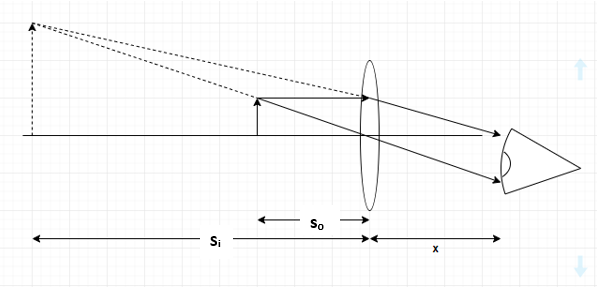# Problem: Anna holds a 14 D magnifier directly in front of her eye to get a close look at a 19-mm-diameter penny. Assume a typical 25 cm near point and a distance of 1.7 cm between the lens and the retina.What is the closest possible distance that she can hold the coin to have it appear in focus? Express your answer with appropriate units.

###### FREE Expert Solution$\overline{)\begin{array}{rcl}\frac{\mathbf{1}}{{\mathbf{s}}_{\mathbf{o}}}\mathbf{+}\frac{\mathbf{1}}{{\mathbf{s}}_{\mathbf{i}}}& {\mathbf{=}}& \frac{\mathbf{1}}{\mathbf{f}}\end{array}}$

D = 14 m-1

x = 1.7 cm

Image distance is on the left. Thus it is conventionally negative###### Problem Details

Anna holds a 14 D magnifier directly in front of her eye to get a close look at a 19-mm-diameter penny. Assume a typical 25 cm near point and a distance of 1.7 cm between the lens and the retina.

What is the closest possible distance that she can hold the coin to have it appear in focus? Express your answer with appropriate units.